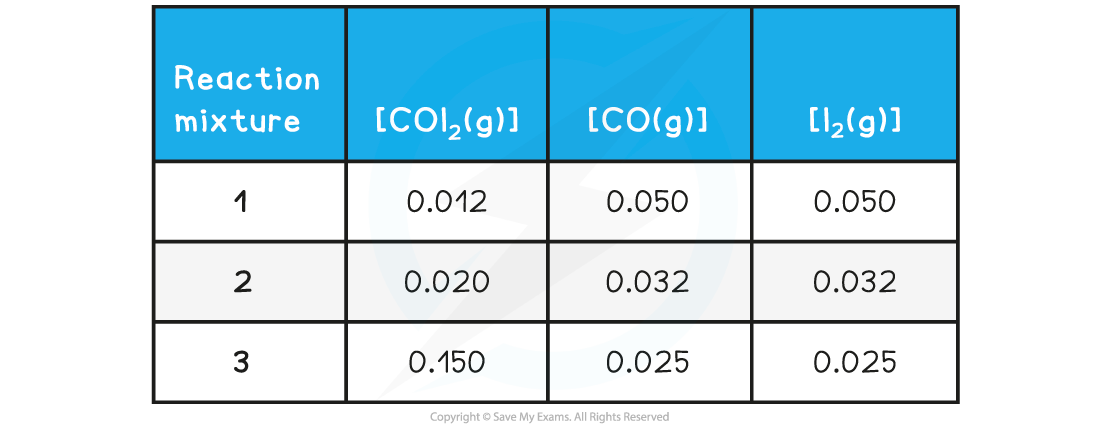# IB DP Chemistry: SL复习笔记7.1.4 The Reaction Quotient

### The Reaction Quotient

• The reaction quotientQ, is the ratio of products and reactants for a reaction that has NOT yet reached equilibrium
• The expression for Q is very similar to Kc:• It is a useful concept because the size of Q can tell us how far a reaction is from equilibrium and in which direction the reaction proceeds
• For Example,
• If Q = Kc then the reaction is at equilibrium, no net reaction occurs
• If Q < Kc the reaction proceeds to the right in favour of the products
• If Q > Kc the reaction proceeds to the left in favour of the reactants
• Using values of the concentrations of the substances present we can work out if a reaction is at equilibrium or not, as the following example shows:

#### Worked Example

The equilibrium constant for the following reaction:

COI2 (g) ⇌ CO (g) + I2 (g)

is 5.1 x 10-2 at 298 K

Deduce whether the following reaction mixture concentrations represent a reaction at equilibrium and for those not at equilibrium indicate the direction is proceeding:The reaction quotient expression isReaction mixture 1:In this mixture Q >> Kc, so Q has to decrease to reach Kc. This means the reaction must be moving to the left, in order to reach equilibrium, so the reactants are favoured

Reaction mixture 2:In this mixture, the value of Q = Kc, so the reaction is at equilibrium

Reaction mixture 3:In this mixture Q < Kc, so Q has to increase to reach Kc. This means the reaction must be moving to the right, in order to reach equilibrium, so the products are favoured

#### Exam Tip

The calculation of Q is not explicitly part of the SL course, just as calculating Kc values only comes in HL chemistry. However, a comparison of Q and Kc is relevant and the worked example is included only to illustrate how Q is determined from experimental data.